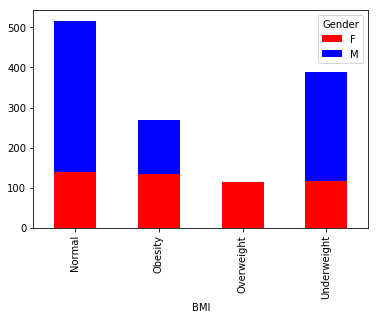# Data Import¶

In :
import matplotlib.pyplot as plt
import pandas as pd


# Stacked Column Chart¶

In :
# Group sum of sales at gender and BMI level
var = df.groupby(['BMI','Gender']).Sales.sum()
var.unstack().plot(kind='bar',stacked=True, color=['red','blue'], grid=False)
#Show stacked column chart
plt.show()In [ ]: